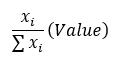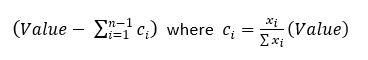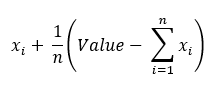# 1.1 - 8.10 - Burst Syntax Elements - Teradata Vantage

## Teradata Vantage™ - Machine Learning Engine Analytic Function Reference

Product
Release Number
1.1
8.10
Published
October 2019
Content Type
Programming Reference
Publication ID
B700-4003-079K
Language
English (United States)
TimeColumn
Specify the names of the InputTable columns that contain the start and end times of the time interval to be burst.
TimeInterval
[Optional] Specify exactly one of TimeIntervalTable, TimeInterval, or NumPoints.
Specify the length of each burst time interval. This value must be either INTEGER or DOUBLE PRECISION.
TargetColumns
Specify the names of InputTable columns to copy to the output table.
TimeDataType
[Optional] Specify the data type of the output columns that correspond to the input table columns that TimeColumn specifies (start_time_column and end_time_column).

If you omit this syntax element, the function infers the data type of start_time_column and end_time_column from the input table and uses the inferred data type for the corresponding output table columns.

If you specify this syntax element, the function can transform the input data to the specified output data type only if both the input column data type and the specified output column data type are in this list:
• INTEGER
• BIGINT
• SMALLINT
• DOUBLE PRECISION
• NUMERIC
• NUMERIC(n[,m])
ValueDataType
[Optional] Specify the data types of the output columns that correspond to the input table columns that TargetColumns specifies.

If you omit this syntax element, the function infers the data type of each target_column from the input table and uses the inferred data type for the corresponding output table column.

If you specify ValueDataType, it must be the same size as TargetColumns. That is, if TargetColumns specifies n columns, ValueDataType must specify n data types. For i in [1, n], value_column_i has value_type_i. However, value_type_i can be empty; for example:

```TargetColumns ('c1', 'c2', 'c3')
ValueDataType (INTEGER, ,VARCHAR)```
If you specify this syntax element, the function can transform the input data to the specified output data type only if both the input column data type and the specified output column data type are in this list:
• INTEGER
• BIGINT
• SMALLINT
• DOUBLE PRECISION
• NUMERIC
• NUMERIC(n[,m])
StartTime
[Optional] Specify the start time for the time interval to be burst.
Default: value in start_time_column
EndTime
[Optional] Specify the end time for the time interval to be burst.
Default: value in end_time_column
NumPoints
[Optional] Specify exactly one of TimeIntervalTable, TimeInterval, or NumPoints.

Specify the number of data points in each burst time interval. This value must be an INTEGER.

ValuesBeforeFirst
[Optional] Specify the values to use if start_time is before start_time_column. Each of these values must have the same data type as its corresponding target_column. Values of data type VARCHAR are case-insensitive.
If you specify ValuesBeforeFirst, it must be the same size as TargetColumns. That is, if TargetColumns specifies n columns, ValuesBeforeFirst must specify n values. For i in [1, n], value_column_i has the value before_first_value_i. However, before_first_value_i can be empty; for example:
```TargetColumns ('c1', 'c2', 'c3')
ValuesBeforeFirst (1, ,'abc')```
If before_first_value_i is empty, value_column_i has the value NULL.
Default: value_column_i has the value NULL for i in [1, n].
ValuesAfterLast
[Optional] Specify the values to use if end_time is after end_time_column. Each of these values must have the same data type as its corresponding target_column. Values of data type VARCHAR are case-insensitive.
If you specify ValuesAfterLast, it must be the same size as TargetColumns. That is, if TargetColumns specifies n columns, ValuesAfterLast must specify n values. For i in [1, n], value_column_i has the value after_last_value_i. However, after_last_value_i can be empty; for example:
```TargetColumns ('c1', 'c2', 'c3')
ValuesAfterLast (1, ,'abc')```
If after_last_value_i is empty, value_column_i has the value NULL.
Default: value_column_i has the value NULL for i in [1, n].
SplitCriteria
[Optional] Specify how to split target_column values into subintervals:
Option Description
'nosplit' (Default) The function assigns to each subinterval the sum of the values in the rows in that interval. See Burst Example: TimeInterval, SplitCriteria ('nosplit') and Burst Example: TimeIntervalTable File (where SplitCriteria defaults to 'nosplit').
'proportional' The function does the following:
1. Determines the number of subintervals for each row.
2. Divides the target_column values evenly across all subintervals.
3. Adds the contributions from all rows to each subinterval.
'random' The function does the following:
1. Determines the number of subintervals for each row.
2. Draws x i for each subinterval i.

The distribution is uniform on (0,1). The value assigned to each subinterval except the last is:where Value is the value of the input table for that row.

The value assigned to the last subinterval is:3. Adds the contributions from all rows to each subinterval.
'gaussian' The function does the following:
1. Determines the number of subintervals for each row.
2. Draws x i for each subinterval i.

The distribution is a standard normal distribution. The value assigned to each subinterval except the last, and the value assigned to the last subinterval, are calculated with the equations shown for 'random'.

3. Adds the contributions from all rows to each subinterval.
'poisson' Use 'poisson' only if the means of each target_column is nonnegative.

For data sets with an expected mean value greater than 40, Teradata recommends selecting 'gaussian' instead of 'poisson'. The performance of the 'poisson' option decreases as the mean of the data set increases.

The function does the following:
1. Determines the number of subintervals for each row.
2. Draws x i for each subinterval i.

The distribution is a Poisson distribution with mean:

Value / number_of_subintervals

Each subinterval is assigned this value:3. Adds the contributions from all rows to each subinterval.
Seed
[Optional] Use only if SplitCriteria is 'random' or 'gaussian'. Specify the value for initializing the random number generator the algorithm uses for repeatable results.
For repeatable results, use both the Seed and UniqueID syntax elements. For more information, see Nondeterministic Results and UniqueID Syntax Element.

Default: 0

Accumulate
[Optional] Specify the names of InputTable columns (other than those specified by TimeColumn and TargetColumns) to copy to the output table.
Default behavior: The function copies to the output table only the columns specified by TimeColumn and TargetColumns.